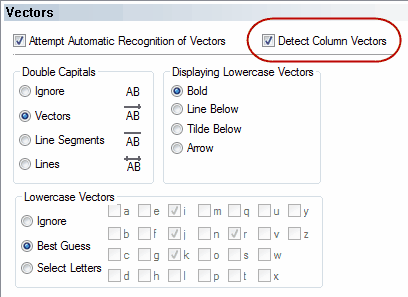﻿ Column Vectors (COPY)

# Column Vectors

Top  Previous  Next

If you wish to enter vectors in the vertical format, they are simply entered as (3,2) using a comma as a separator.  Three-dimensional vectors are entered in the same way.  If you normally use a comma as a decimal point, the equation tool will automatically detect this and you must use a semicolon to separate components of a vertical vector.

## Examples: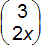is entered as (3,2x) or (3;2x)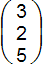is entered as (3,2,5) or (3;2;5)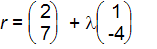is entered as r=(2,7)+la(1,-4) or r=(2;7)+la(1;-4)

You must have selected the Detect Column Vectors option to allow the equation tool to detect column vectors and matrices.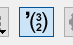## or